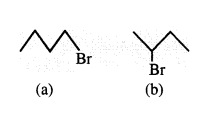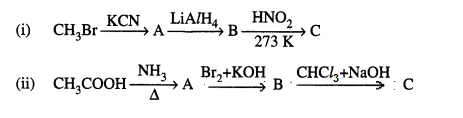## CHEMISTRY (Theory)

### 2014

[Time allowed: 3hrs] [Maximum Marks:70]

General Instructions :
(i)   All questions are compulsory.
(ii)  Questions numbers 1 to 8 are very short-answer questions and carry 1 mark each.
(iii) Questions numbers 9 to 18 are also short-answer questions and carry 2 marks each.
(iv)  Questions numbers 19 to 27 are long-answer questions and carry 3 marks each.
(v)   Questions numbers 28 to 30 are long-answer questions and carry 5 marks each.
(vi)  Use Log Tables,if neccessary.Use of calculators is not allowed.

 1. Give one example of sol and gel? 1 2. Which reducing agent is employed to get copper from the leached low grade copper ore? 1 3. Write the IUPAC name of the compound 1 CH3 - CH - CH2 - CHO                            NH2 4. Which of the following isomers is more volatile : 1 o-nitrophenol or p-nitrophenol ? 5. Some liquids on mixing form 'azeotropes'. What are 'azeotropes' ? 1 6. Arrange the following in increasing order of basic strength : 1 C6H5NH2, C6H5NHCH3, C6H5CH2NH2

 7. Which component of starch is a branched polymer of o-glucose and insoluble in water? 1 8. Which of the following is more stable complex and why ? 1 [Co(NH3)6]3+ and [Co(en)3]3+ 9. State Henry's law. What is the effect of temperature on the solubility of a gas in a liquid ? 2 10. Define the following terms : 2 (i) Pseudo fust order reaction (ii) Half life period of reacrion (t½). 11. Write the principle behind the following methods of refining : 2 (i) Hydraulic washing (ii) Vapour phase refining 12. Draw the structure of major monohalo product in each of the following reactions : 2 (i) (ii)13. (i) Which alkyl halide from the following pair is chiral and undergoes faster SN2 reaction ? 2(ii) Out of SN1 and SN2, which reaction occurs with (a) Inversion of configuration (b) Racemisation 14. Complete the following chemical equations': 2 (i) Ca3Pr2 + H2O → (ii) Cu + H2SO4(conc.) → OR Arrange the following in the order of property indicated against each set : (i) HF, HCl, HBr, HI - increasing bond dissociation enthalpy. (ii) H2O, H2S, H2Se, H2Te - increasing acidic character 15. Write the IUPAC name of the complex [Cr(NH3)4Cl2]+. What type of isomerism does it exhibit ?
 16 An element with density 11.2 g cm-3 forms a f.c.c. lattice with edge length of 4x l0-8 cm. Calculate the atomic mass of the element. 2

(Given : NA = 6.022x 1023 mol-l)
17.
Examine the given defective crystal
2
A+   B-   A+   B-   A+
B-   O    B-     A+   B-
A+   B-   A+   O     A+
B-   A+    B-    A+   B-
(i) What type of stoichiometric defect is shown by the crystal ?
(ii) How is the density of the crystal affected by this defect ?
(iii) What type of ionic substances show such defect ?
18.
Calculate the mass of compound (molar mass = 256 gmol-1) to be dissolved benzene to lower its freezing point
2
by 0.48 K (Kf = 5.12 K kg mol-l).
19.
Give the structures of A, B and C in the following reactions :
3OR
How will you convert the following :
(i) Nitrobenzene into aniline
(ii) Ethanoic acid into methanamine
(iii) Aniline into N-phenylethanamide
(Write the chemical equations involved.)
 20. (a) Write the mechanism of the following reaction : 3(b) Write the equation involved in Reimer-Tiemann reaction. 21. After the ban on plastic bags, students of one school decided to make the people aware of the harmful effects of 3 plastic bags on environment and yamuna River. To make the awareness more impactful, they organized rally by joining hands with other schools and distributed paper bags to vegetable vendors,shopkeepers and departmental stores. All students pledged not to use polythene bags in future to save yamuna River. After reading the above passage, answer the foilowing questions : (i) What values are shown by the students ? (ii) what are biodegradable polymers ? Give one example. (iii) Is polythene a condensation or an addition polymer ? 22. (a) Draw the structures of the following : 3 (i) XeF2 (ii) BrF3 (b) write the structural difference between white phosphorus and red phosphorus. 23. (a) In reference to Freundlich adsorption isotherm write the expression for adsorption of gases on solids in the 3 form of an equation. (b) write an important characteristic of lyophilic sols. (c) Based on type of particles of dispersed phase, give one example each of associated colloid and multimolecular colloid. 24. Account for the following : 3 (i) Bi(V) is a stronger oxidizing agent than Sb(V). (ii) N - N single bond is weaker than P - P single bond. (iii) Noble gases have very low boiling points.
 25. (i) Name the sweetening agent used in the preparation of sweets for a diabetic patient. 3 (ii) What are antibiotics ? Give an example. (iii) Give two examples of macromolecules that are chosen as drug targets. 26. The following data were obtained during the first order thermal decomposition of SO2Cl2 at a constant volume : 3 SO2Cl2(g) → SO2(g) + Cl2(g)
Experiment Time/s-1 Total pressure/atm
1 0 0.4
2 100 0.7
 Calculate the rate constant. (Given : log4 = 0.6021,1og2 = 0.3010) 27. (i) Deficiency of which vitamin causes rickets ? 3 (ii) Give an example for each of fibrous protein and globular protein. (iii) write the product formed on reaction of D-glucose with Br2 water. 28. (a) Write the products of the following reactions : 3,2(b) Give simple chemical tests to distinguish between the following pairs of compounds: (i) Benzaldehyde and Benzoic acid (ii) Propanal and Propanone
 (a) Account for the following : (i) CH3CHO is more reactive than CH3COCH3 towards reaction with HCN. (ii) Carboxylic acid is a stronger acid than phenol. (b) Write the chemical equations to illustrate the following name reactions : (i) Wolff-Kishner reduction (ii) Aldolcondensation (iii) Cannizzaro reaction 29. (a) Define the following terms : 3,2 (i) Limiting molar conductivity (ii) Fuel cell (b) Resistance of a conductivity cell filled with 0.1 mol L-l KCl solution is 100 Q. If the resistance of the same cell when filled with 0.02 mol tr KCL solution is 520 dr, calculate the conductivity and molar conductivity of 0.02 mol L-1 KC/ solution. The conductivity of 0.1 mol L-l KC/ solution is 1.29 x lla Cl-l cm-l. OR (a) State Faraday's first law of elecrolysis. How much charge in terms of Faraday is required for the reduction of 1 mol of Cu2+ to Cu. (b) Calculate emf of the following cell at298K: Mg(s) | Mg2+(0.1M) || Cu2+(0.01) | cu(s) [Given Eocell = +2.71V, 1 F = 96500 C mol-l] 30. (a) How do you prepare : 5 (i) K2MnO4 from MnO2 ? (ii) Na2Cr2O7 from Na2CrO4 ? (b) Account for the following : (i) Mn2+ is more stable than Fe2+ towards oxidation to +3 state (ii) The enthalpy of atomization is lowest for Zn in 3d series of the transition elements (iii) Actinoid elements show wide range of oxidation states. OR (i) Name the element of 3d transition series which shows maximum number of oxidation states. Why does it show so ? (ii) Which transition metal of 3d series has positive Eo(M2+/M) value and why ? (iii) Out of Cr3+ and Mn3+, which is a stronger oxidizing agent and why ? (iv) Name a member of the lanthanoid series which is well known to exhibit +2 oxidation state. (v) Complete the following equation : MnO-4 + 8H+ + 5e-→